# Dispersive Material Property

## General Usage

 CSX = AddLorentzMaterial(CSX, name, varargin)


with the following parameters:

• CSX: The original CSX structure
• name: name of the property
• varargin: a list of variable arguments

To define the material properties use:

 CSX = SetMaterialProperty(CSX, name, varargin)


with the following parameters:

• CSX: The original CSX structure
• name: name of this property (same as above!)
• varargin: a list of variable arguments to define the properties:

#### Conventional material parameters:

• Epsilon: relative electric permittivity for$f \to \infty$ (must be >=1)
• Mue: relative magnetic permeability for$f \to \infty$ (must be >=1)
• Kappa: electric conductivity (must be >=0)
• Sigma: magnetic conductivity (non-physical property, must be >=0)

#### Drude material parameters:

• EpsilonPlasmaFrequency: electric plasma frequency (fplasma,1, see below)
• MuePlasmaFrequency: magnetic plasma frequency
• EpsilonRelaxTime: electric plasma relaxation time (τp, losses, see below)
• MueRelaxTime: magnetic plasma relaxation time (losses)

#### Drude higher order parameter (p>1):

• EpsilonPlasmaFrequency_p: p-th order electric plasma frequency (fplasma,p, see below)
• MuePlasmaFrequency_p: p-th order magnetic plasma frequency
• EpsilonRelaxTime_p: p-th order electric plasma relaxation time (losses)
• MueRelaxTime_p: p-th order magnetic plasma relaxation time (losses)

#### Lorentz material parameters:

Note: Available only for openEMS >= v0.0.31!!

In addition to the drude parameter, the Lorentz-pole frequency can be defined:

• EpsilonLorPoleFrequency: first electric Lorentz pole frequency (fLor,1, see below)
• EpsilonLorPoleFrequency_p: p-th electric Lorentz pole frequency (fLor,p, see below)
• MueLorPoleFrequency: first magnetic Lorentz pole frequency
• MueLorPoleFrequency_p: p-th magnetic Lorentz pole frequency

## Drude Material

### Physical Model & Parameter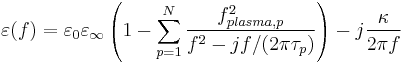$\varepsilon(f) = \varepsilon_0\varepsilon_\infty \left( 1 - \sum_{p=1}^N \frac{f_{plasma,p}^2}{f^2-j f/(2\pi\tau_p)}\right) - j\frac{\kappa}{2\pi f}$

with the parameter:

•$\varepsilon_\infty$ the relative permittivity for$f \to \infty$
• κ the electric conductivity
• fplasma,p the p-th "plasma" frequency
• τp the p-th relaxation time (damping)

### Example

CSX = AddLorentzMaterial(CSX,'drude');
CSX = SetMaterialProperty(CSX,'drude','Epsilon',eps_r,'Kappa',kappa);
CSX = SetMaterialProperty(CSX,'drude','EpsilonPlasmaFrequency', 5e9, 'EpsilonRelaxTime', 5e-9);


## Lorentz Material

### Physical Model & Parameter$\varepsilon(f) = \varepsilon_0\varepsilon_\infty \left( 1 - \sum_{p=1}^N \frac{f_{plasma,p}^2}{f^2-f_{Lor,p}^2-jf/(2\pi\tau_p)}\right) - j\frac{\kappa}{2\pi f}$

with the parameter:

•$\varepsilon_\infty$ the relative permittivity for$f \to \infty$
• κ the electric conductivity
• fplasma,p the p-th drude "plasma" frequency
• fLor,p the p-th Lorentz pole frequency
• τp the p-th relaxation time (damping)

### Example

CSX = AddLorentzMaterial(CSX,'lorentz');
CSX = SetMaterialProperty(CSX,'lorentz','Epsilon',eps_r,'Kappa',kappa);
CSX = SetMaterialProperty(CSX,'lorentz','EpsilonPlasmaFrequency', 5e9, 'EpsilonLorPoleFrequency', 10e9, 'EpsilonRelaxTime', 5e-9);


## Relation to other formulations

•$\varepsilon(\omega) = \varepsilon_0\varepsilon_\infty + \varepsilon_0 \sum_{p=1}^N \frac{(\varepsilon_{dc,p}-\varepsilon_\infty)\omega^2_{p}}{\omega_{p}^2+2j\omega\delta_p-\omega^2}$

Conversion:$f_{Lor,p} = \frac{\omega_{p}}{2\pi}$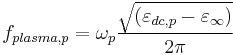$f_{plasma,p} = \omega_{p}\frac{\sqrt{(\varepsilon_{dc,p}-\varepsilon_\infty)}}{2\pi}$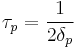$\tau_p = \frac{1}{2\delta_p}$

κ = 0

Researchers in Physics often adopt a different sign convention, in which they use e iωt for time-harmonic quantities rather than eiωt in engineering. Therefor in some textbook the Lorentz model is: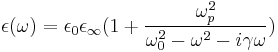$\epsilon(\omega) = \epsilon_0\epsilon_{\infty} (1+ \frac{\omega_p^2}{\omega_0^2 - \omega^2 - i\gamma \omega})$

And the Drude model is: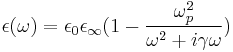$\epsilon(\omega) = \epsilon_0\epsilon_{\infty} (1- \frac{\omega_p^2}{\omega^2 + i\gamma \omega})$

Where ωp is the plasma frequency and ω0 is the plasmonic resonant frequency and γ represents the damping effect in material.